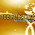## Wednesday, 30 January 2013

### C PROGRAM: TO CALCULATE ARITHMETIC MEAN OF EACH ROW OF MATRIX

CODING:
#include<stdio.h>
#include<conio.h>
void main()
{
int a,m,n,i,j,sum;
double am;
clrscr();
printf("Enter order of matrix ");
scanf("%d%d",&m,&n);
printf("Enter values of matrix\n");
for(i=1;i<=m;i++)
{
sum=0;
for(j=1;j<=n;j++)
{
printf("Enter value of a[%d][%d] ",i,j);
scanf("%d",&a[i][j]);
sum=sum+a[i][j];
}
am[i]=(double)sum/n;
}
for(i=1;i<=m;i++)
{
printf("\nArithmetic Mean of row %d = %f",i,am[i]);
}
getch();
}CODINGOUTPUT

For any query or suggestion please comment below...

1.1.